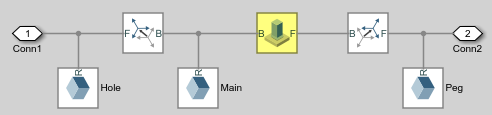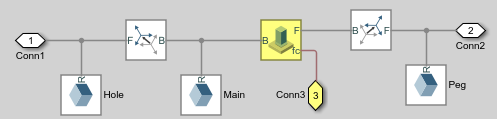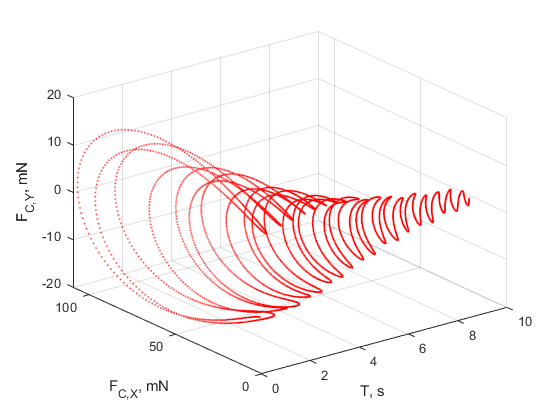## Sense Constraint Forces

### Model Overview

Simscape™ Multibody™ provides various types of force and torque sensing. Using joint blocks, you can sense the actuation forces and torques driving individual joint primitives. You can also sense the total and constraint forces acting on an entire joint.

In this tutorial, you use a Weld Joint block to sense the time-varying internal forces that hold a body together. A double-pendulum model, `smdoc_double_pendulum`, provides the starting point for the tutorial. For information on how to create this model, see Model an Open-Loop Kinematic Chain.By connecting the Weld Joint block between solid elements in a binary link subsystem, you can sense the constraint forces acting between them. The following figure shows the constraint forces that you sense in this tutorial. The longitudinal constraint force aligns with the X axis of the weld joint frames. The transverse constraint force aligns with the Y axis. The constraint force along the Z axis is negligible and therefore ignored.The Weld Joint block enables you to sense the constraint force that the follower frame exerts on the base frame or, alternatively, the constraint force that the base frame exerts on the follower frame. The two forces have the same magnitude but, as shown in the binary link schematic, opposite directions. In this tutorial, you sense the constraint force that the follower frame exerts on the base frame.

You can also select the frame to resolve the constraint force measurement in. The resolution frame can be either the base frame or the follower frame. Certain joint blocks allow their port frames to have different orientations, causing the same measurement to differ depending on your choice of resolution frame. However, because the Weld Joint block provides zero degrees of freedom, both resolution frames yield the same constraint force vector components.

### Add Weld Joint Block to Model

1. At the MATLAB® command prompt, enter `smdoc_double_pendulum`. A double-pendulum model opens up.

2. Click the Look Inside Mask arrow in the Binary Link A1 subsystem block.

3. From the Simscape > Multibody > Joints library, drag a Weld Joint block.

4. Connect the Weld Joint block as shown in the figure. This block enables you to sense the constraint forces that hold the body together during motion. Because it provides zero degrees of freedom between its port frames, it has no effect on model dynamics.### Add Constraint Force Sensing

1. In the Weld Joint block dialog box, select Constraint Force. The block exposes a physical signal output port labeled fc.

2. Add a Simscape Output port to the subsystem block diagram. Connect the block as shown in the figure and exit the subsystem view.3. Drag the following blocks into the main window of the model. These blocks enable you to output the constraint force signal into the MATLAB workspace.

LibraryBlock
Simscape > UtilitiesPS-Simulink Converter
Simulink > SinksTo Workspace

4. Connect the blocks as shown in the figure. Check that the PS-Simulink Converter block connects to the newly added Simscape port.5. Specify these block parameters.

BlockDialog Box ParameterValue
PS-Simulink ConverterOutput signal unit`mN`
To WorkspaceVariable name`fcf_weld`

Units of `mN` are appropriate for this model, which contains Aluminum links roughly 30 cm × 2 cm × 0.8 cm.

### Add Damping to Joints

In each Revolute Joint block dialog box, select Internal Mechanics > Damping Coefficient and enter `1e-5`. The damping coefficient enables you to model energy dissipation during motion, so that the double-pendulum model eventually comes to rest.

### Simulate Model

1. In the Modeling tab, click .

2. In the Solver tab of the Configuration Parameters window, set the Solver parameter to `ode15s`. This is the recommended solver for physical models.

3. In the same tab, set the Max step size parameter to `0.001` s.

4. Run the simulation. Mechanics Explorer opens with a dynamic view of the model. In the Mechanics Explorer menu bar, select the Isometric View button to view the double pendulum from an isometric perspective.### Plot Constraint Forces

At the MATLAB command prompt, enter the following plot commands:

```figure; plot3(fcf_weld.time, fcf_weld.data(:,1), fcf_weld.data(:,2),... '.', 'MarkerSize', 1, 'Color', 'r'); grid on; xlabel('T, s'); ylabel('F_{C,X}, mN'); zlabel('F_{C,Y}, mN'); ```
MATLAB plots the axial and transverse constraint forces with respect to time in 3-D. The figure shows the resulting plot.